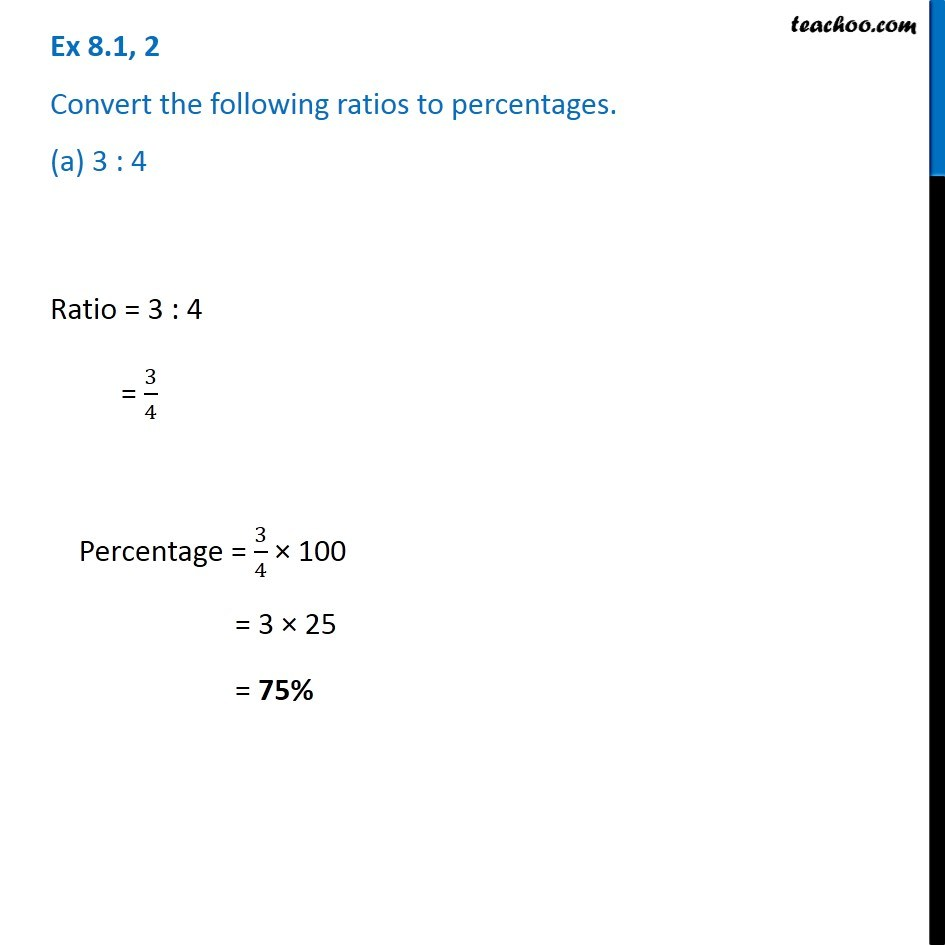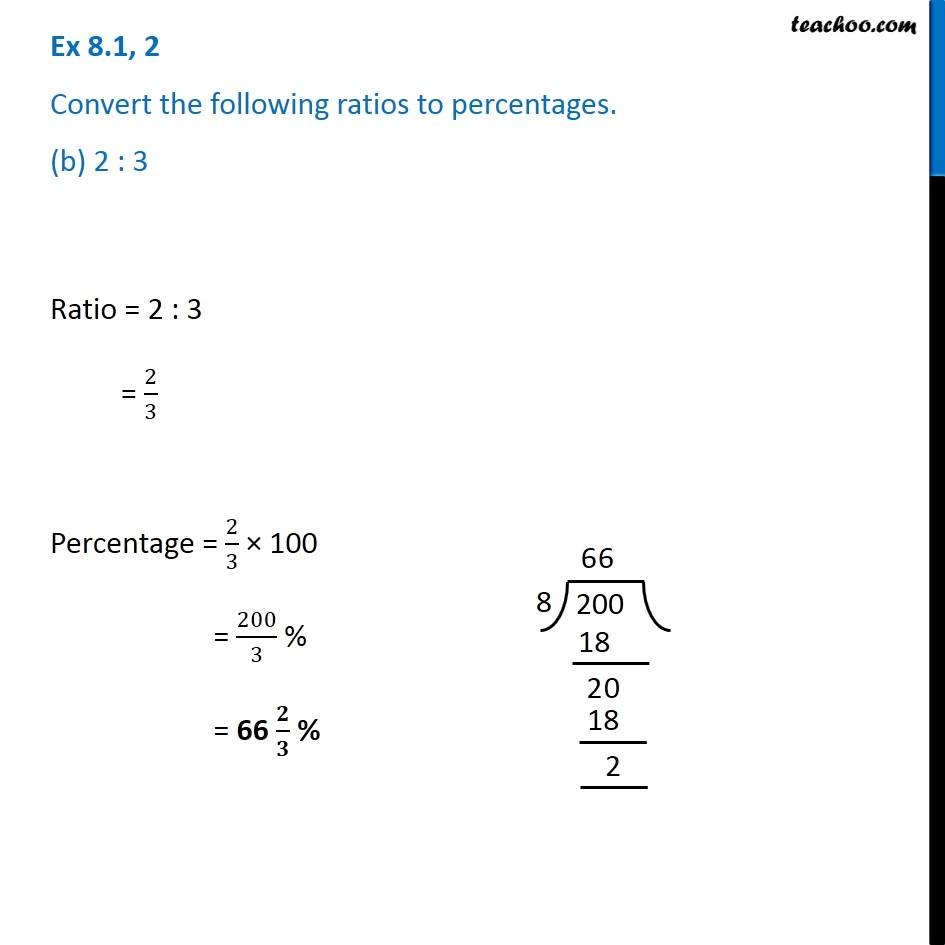Subscribe to our Youtube Channel - https://you.tube/teachoo

1. Chapter 8 Class 8 Comparing Quantities
2. Serial order wise
3. Ex 8.1

Transcript

Ex 8.1, 2 Convert the following ratios to percentages. (a) 3 : 4Ratio = 3 : 4 = 3/4 Percentage = 3/4 × 100 = 3 × 25 = 75% Ex 8.1, 2 Convert the following ratios to percentages. (b) 2 : 3Ratio = 2 : 3 = 2/3 Percentage = 2/3 × 100 = 200/3 % = 66 𝟐/𝟑 %

Ex 8.1